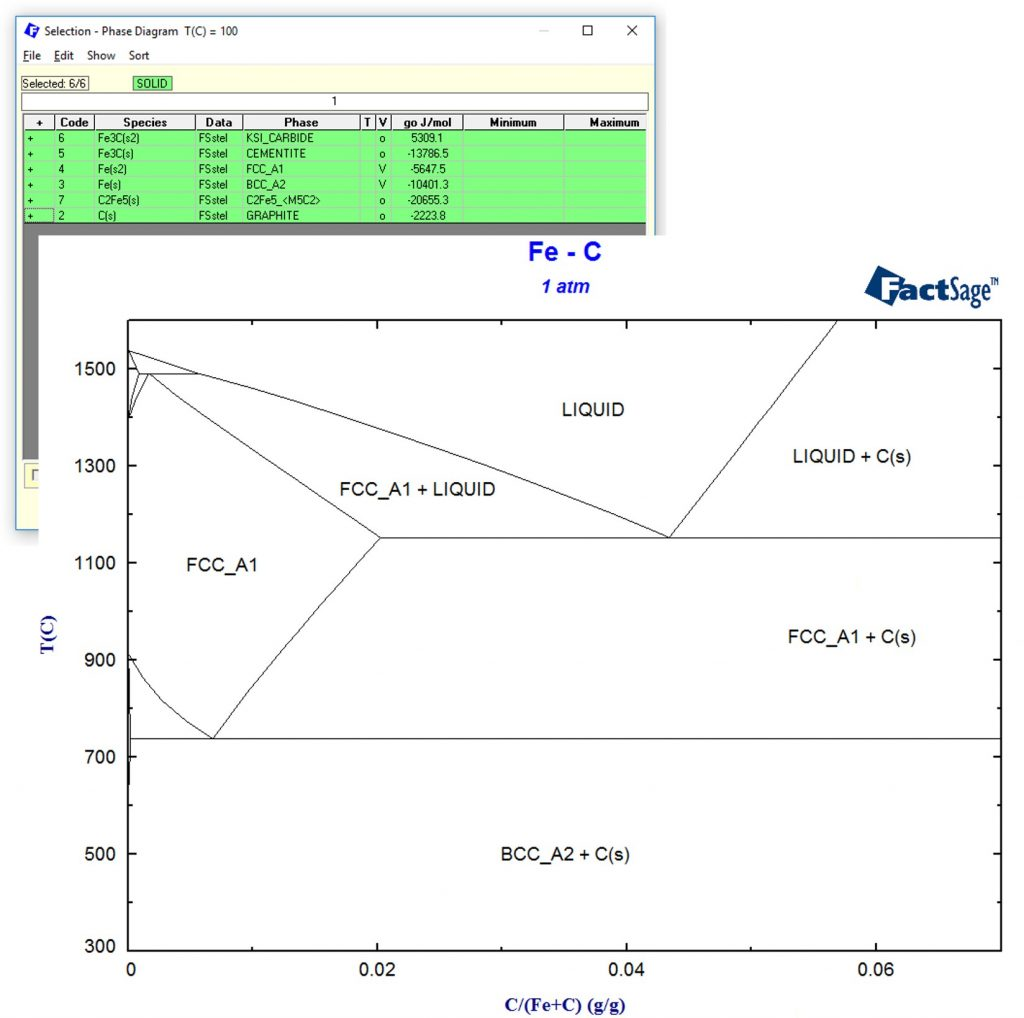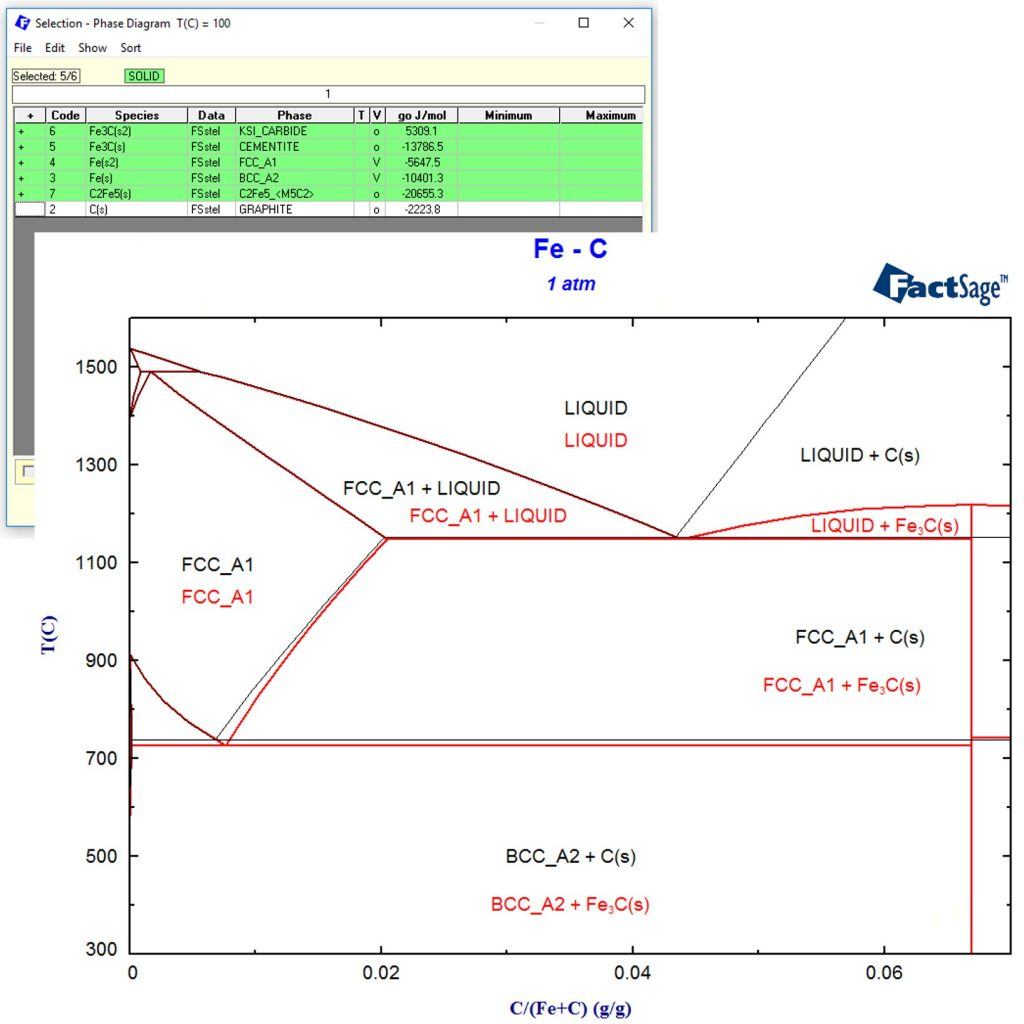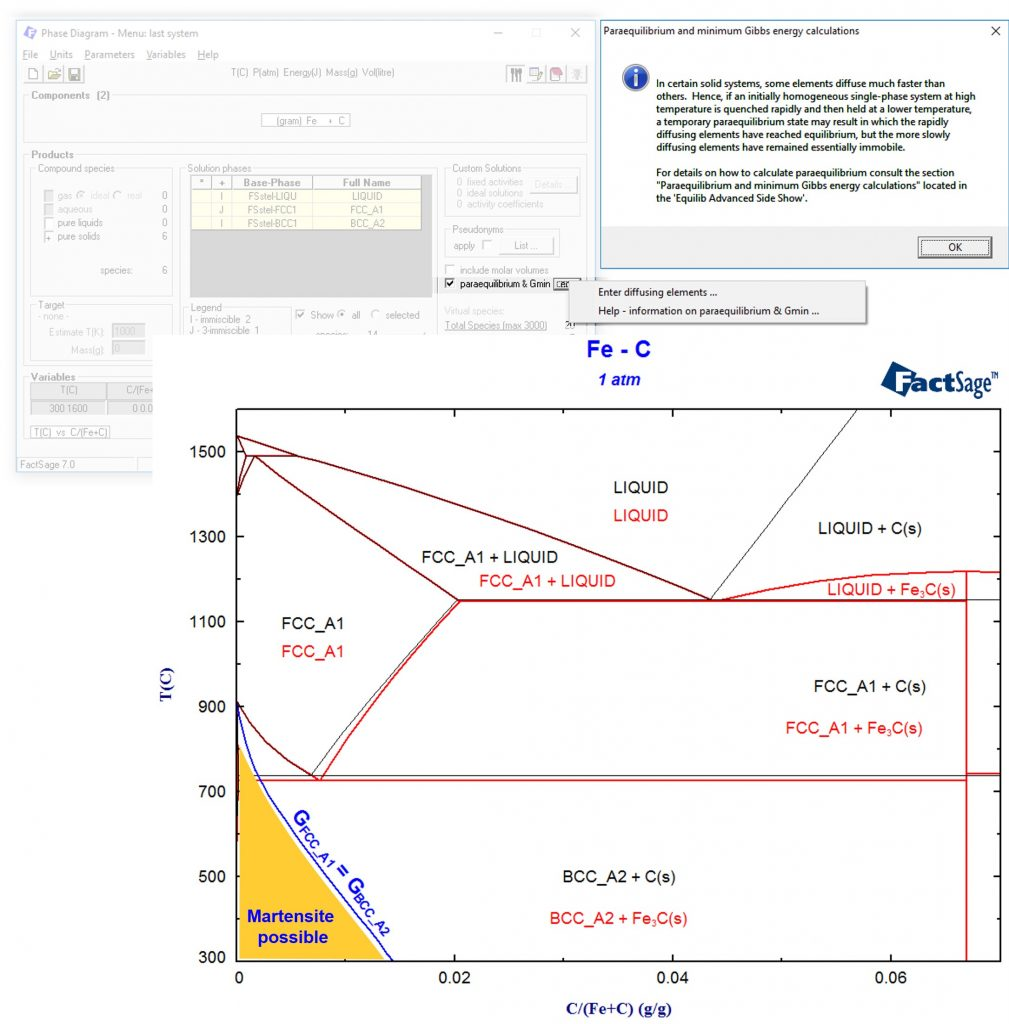# Example for a FactSage calculation of a non-equilibrium process

It is a common misconception that thermodynamic calculations can only be used for processes that are in equilibrium. In fact, there is a lot to learn from an Integrated Thermodynamic Databank System like FactSage even if you are far away from equilibrium! As example we consider the popular Fe-C system: If you choose all phases in the FactSage Steel database and calculate the phase diagram, this is what you get:For low carbon contents, it is a well-known fact that Graphite C(s) does not form due to kinetic restrictions. Therefore, we simply remove Graphite C(s) from the selection of possible products and recalculate the phase diagram. This is shown in the following phase diagram in red as an overlay of the previous phase diagram.Even though it looks similar to the phase diagram above, this is a completely different phase diagram since it takes kinetic limitations into account! If the user wants to obtain thermochemical information of a phase that is not allowed to form during a calculation, it is also possible to set that phase “dormant”. Thus in the current example, activity of Carbon can be calculated, even though Carbon is not allowed to precipitate as graphite.

We can even go further away from thermodynamic equilibrium by completely suppressing diffusion, meaning that the single phase with lowest Gibbs energy is formed. These calculations can be activated by using the “paraequilibrium & Gmin” option. The result is a single line where the Gibbs energies of the austenite (FCC) and ferrite (BCC) are equal. This line is integrated in blue into the phase diagram:Martensite is a body-centered tetragonal crystal structure (BCT), that is similar in Gibbs energy to the BCC phase but less stable (otherwise it would be present in the phase diagram). Since the martensite formation is a diffusionless transformation, we have now calculated an upper bound for the marteniste start temperature: Only below the blue line it will be possible to form martensite!

For multicomponent systems it would also be possible to allow one element to be mobile while the others are not, e.g. for Fe-Cr-C steels to calculate a metastable phase formation diagram where only carbides are allowed to form that exhibit the same Fe/Cr ratio as the matrix.

Interestingly, even highly non-equilibrium processes such as vapor phase condensation can be modelled on the basis of a paraequilibrium, please check the following two open access publications:

Chang et al., Modeling of metastable phase formation diagrams for sputtered thin films. Science and Technology of Advanced Materials 17, 210 (2016).

to Baben et al., Unprecedented thermal stability of inherently metastable titanium aluminum nitride by point defect engineering. Materials Research Letters (2016).

This site uses Akismet to reduce spam. Learn how your comment data is processed.